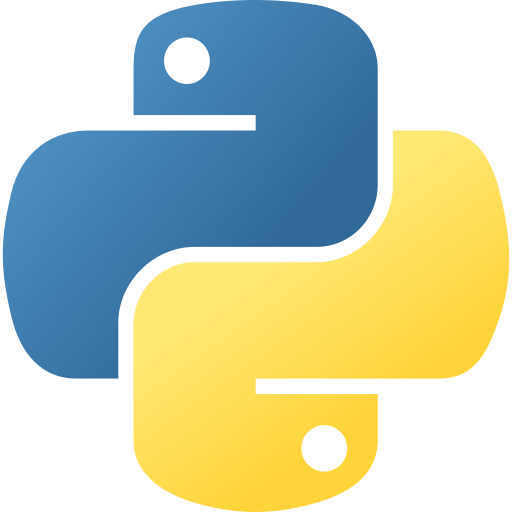# AI coding assistant for busy programmers

## Compute the first five Fibonacci numbers with a O(2) complexity. in Python## 🤖 Code Explanation

This code calculates and prints the first 5 Fibonacci numbers. Fibonacci numbers are a sequence of numbers where each number is the sum of the previous two.